Home    |    Teacher    |    Parents    |    Glossary    |    About UsOne way to think of a fraction is as a division that hasn't been done yet. Why do we even use fractions? Why don't we just divide the two numbers and use the decimal instead? In this day of cheap calculators, that's a very good question. Fractions were invented long before decimal numbers, as a way of showing portions less than 1, and they're still hanging around. They're used in cooking, in building, in sewing, in the stock market - they're everywhere, and we need to understand them.

Just to review, the number above the bar is called the numerator, and the number below the bar is called the denominator.

We can read this fraction as three-fourths, three over four, or three divided by four.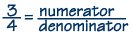Every fraction can be converted to a decimal by dividing. If you use the calculator to divide 3 by 4, you'll find that it is equal to 0.75.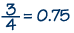Here are some other fractions and their decimal equivalents. Remember, you can find the decimal equivalent of any fraction by dividing.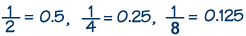Here are some terms that are very important when working with fractions.

Proper fraction
When the numerator is less than the denominator, we call the expression a proper fraction. These are some examples of proper fractions.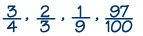Improper fraction
An improper fraction occurs when the numerator is greater than or equal to the denominator. These are some examples of improper fractions: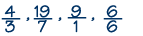Mixed number
When an expression consists of a whole number and a proper fraction, we call it a mixed number. Here are some examples of mixed numbers: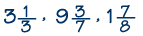We can convert a mixed number to an improper fraction. First, multiply the whole number by the denominator of the fraction. Then, add the numerator of the fraction to the product. Finally, write the sum over the original denominator. In this example, since three thirds is a whole, the whole number 1 is three thirds plus one more third, which equals four thirds.

Convert 1-1/3 to an improper fraction: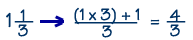Equivalent fractions
There are many ways to write a fraction of a whole. Fractions that represent the same number are called equivalent fractions. This is basically the same thing as equal ratios. For example, ½, 2/4, and 4/8 are all equivalent fractions. To find out if two fractions are equivalent, use a calculator and divide. If the answer is the same, then they are equivalent.

Reciprocal
When the product of two fractions equals 1, the fractions are reciprocals. Every nonzero fraction has a reciprocal. It's easy to determine the reciprocal of a fraction since all you have to do is switch the numerator and denominator--just turn the fraction over. Here's how to find the reciprocal of three-fourths.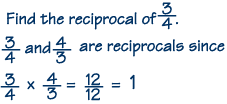To find the reciprocal of a whole number, just put 1 over the whole number. For example, the reciprocal of 2 is ½.

 Homework Help | Pre-Algebra | FractionsEmail this page to a friendSearch·  Definitions·  Reducing fractions·  Adding and subtract-     ing fractions·  Multiplying fractions·  Dividing fractions·  Adding and subtract-     ing mixed numbers·  Multiplying     mixed numbers·  Dividing     mixed numbersFirst Glance In Depth Examples WorkoutFraction definitions